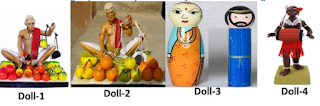### CBSE Class 10 Maths Basic Term 2 Sample Paper Solutions 2021-22

CLASS 10 CBSE
Maths Basic 2021-22

Section A
Find the roots of the quadratic equation 3x^2 − 7x − 6 = 0.

Find the values of k for which the quadratic equation 3x^2 + kx + 3 = 0 has real and equal roots.

Three cubes each of volume 64"cm"^3 are joined end to end to form a cuboid. Find the total surface area of the cuboid so formed?

An inter house cricket match was organized by a school. Distribution of runs made by the students is given below. Find the median runs scored.

Find the common difference of the AP 4,9,14,… If the first term changes to 6 and the common difference remains the same then write the new AP.

The mode of the following frequency distribution is 38. Find the value of x.

Section C
An AP 5, 8, 11…has 40 terms. Find the last term. Also find the sum of the last 10 terms.

A tree is broken due to the storm in such a way that the top of the tree touches the ground and makes an angle of 300 with the ground. Length of the broken upper part of the tree is 8 meters. Find the height of the tree before it was broken.

Two poles of equal height are standing opposite each other on either side of the road 80m wide. From a point between them on the road the angles of elevation of the top of the two poles are respectively 600 and 300 . Find the distance of the point from the two poles.

The sum of the squares of three positive numbers that are consecutive multiples of 5 is 725. Find the three numbers.

Section C
Construct two concentric circles of radii 3cm and 7cm. Draw two tangents to the smaller circle from a point P which lies on the bigger circle.
Draw a pair of tangents to a circle of radius 6cm which are inclined to each other at an angle of 60^0. Also find the length of the tangent.

The following age wise chart of 300 passengers flying from Delhi to Pune is prepared by the Airlines staff.

A lighthouse is a tall tower with light near the top. These are often built on islands, coasts or on cliffs. Lighthouses on water surface act as a navigational aid to the mariners and send warning to boats and ships for dangers. Initially wood, coal would be used as illuminators. Gradually it was replaced by candles, lanterns, electric lights. Nowadays they are run by machines and remote monitoring.

Prongs Reef lighthouse of Mumbai was constructed in 1874-75. It is approximately 40 meters high and its beam can be seen at a distance of 30 kilometres. A ship and a boat are coming towards the lighthouse from opposite directions. Angles of depression of flash light from the lighthouse to the boat and the ship are 30 0 and 600 respectively.

i) Which of the two, boat or the ship is nearer to the light house. Find its distance from the lighthouse?
ii) Find the time taken by the boat to reach the light house if it is moving at the rate of 20 km per hour.

Krishnanagar is a small town in Nadia District of West Bengal. Krishnanagar clay dolls are unique in their realism and quality of their finish. They are created by modelling coils of clay over a metal frame. The figures are painted in natural colours and their hair is made either by sheep’s wool or jute. Artisans make models starting from fruits, animals, God, goddess, farmer, fisherman, weavers to Donald Duck and present comic characters. These creations are displayed in different national and international museums.
Here are a few images (not to scale) of some clay dolls of Krishnanagar.The ratio of diameters of red spherical apples in Doll-1 to that of spherical oranges in Doll-2 is 2:3. In Doll-3, male doll of blue colour has cylindrical body and a spherical head. The spherical head touches the cylindrical body. The radius of both the spherical head and the cylindrical body is 3cm and the height of the cylindrical body is 8cm. Based on the above information answer the following questions:

i) What is the ratio of the surface areas of red spherical apples in Doll-1 to that of spherical oranges in Doll-2.?

ii) The blue doll of Doll-3 is melted and its clay is used to make the cylindrical drum of Doll-4. If the radius of the drum is also 3cm, find the height of the drum.

### Explain the Role of ‘Justice Party’ in Boycotting of Council Elections | bzziii.com

Arun and Vijay are partners in a firm sharing profits and losses in the ratio of 5:1.  Balance Sheet (Extract)  Liabilities Amount (Rs.) Assets Amount (Rs.) Machinery 40,000 If the value of machinery reflected in the balance sheet is overvalued by 33 1/3%, find out the value of Machinery to be shown in the new Balance Sheet:  (A) ₹ 44,000  (B) ₹48,000  (C) ₹ 32,000  (D) ₹30,000  SOLUTION (D) ₹30,000  Explanation: Machinery is overvalued Value by 33 1/3% of 40,000 Here, 33 1/3% as a fraction = \frac{33\frac{1}{3}}{100} Converting the mixed fraction to an improper fraction, we get = \frac{\frac{100}{3}}{100}  = 100/300 Simplifying this, we get = 100/300 = 1/3 = 100/300 of z = 40,000-z Because, 40,000 is overvalued Value, we will minus the real value from 40,000. = "100x"/"300" + "300z"/"300" = 40,000 = "400z"/"300" = 40,000 = 40,000 \times 300/400 = z ∴ z = 30,000 4 5